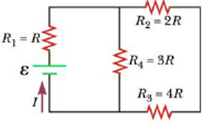Chapter 18, Problem 12P

Chapter
Section
Textbook Problem

Four resistors are connected to a battery as shown in Figure P18.12. (a) Determine the potential difference across each resistor in terms of ε. (b) Determine the current in each resistor in terms of I.Figure P18.12

(a)

To determine
The potential difference across each resistor in terms of ε .

Explanation

Given Info: The resistor in the circuit R2 and R3 is connected in series combination and the resistor R4 and R23 is connected in parallel combination, the total equivalent resistance of R234 is 2R and emf of the battery is ε , The potential difference across the point a and b is mentioned as (ΔVab=2ε/3) and 2R , the equivalent resistance of the resistor R2 and R3 is 6R .

Explanation:

Formula to calculate the equivalent resistance of the resistor R2 and R3 are connected in series is,

R23=R2+R3

• R23 is the equivalent resistance of the resistor R2 and R3 ,
• R2 and R3 is the resistors,

Substitute 2R for R2 and 4R for R3 to find R23 .

R23=2R+4R=6R

Formula to calculate the equivalent resistance of the resistor R4 and R23 are connected in parallel is,

R234=R4R23R4+R23

• R234 is the equivalent resistance of the resistor R4 and R23 ,
• R4 is the resistor,

Substitute 3R for R4 and 6R for R23 to find R234 .

R234=(3R)(6R)(3R+6R)=2R

Thus, the total equivalent resistance of R234 in the given circuit is 2R .

Formula to calculate the total current supplied by the battery is,

I=εR1+R234

• I is the total current,
• ε is the emf of the battery,

Substitute R for R1 and 2R for R234 to find I .

I=εR+2R=ε3R

Formula to calculate the potential difference across the resistor R1 is,

ΔV1=IR1

• ΔV1 is the potential difference across the resistor R1 ,

Substitute R for R1 and ε/3R for I to find ΔV1 .

ΔV1=(ε3R)R=(ε3)

Formula to calculate the potential difference across the resistor R4 is,

ΔV4=IR234

• ΔV4 is the potential difference,

Substitute 2R for R234 and ε/3R for I to find ΔV4 .

ΔV4=(ε3R)(2R)=(2ε3)

Since the potential difference across the resistor R4 is ΔV4 and it is equal to the potential difference across the point a and b is mentioned as ΔVab

(b)

To determine
The current through each resistor.

Still sussing out bartleby?

Check out a sample textbook solution.

See a sample solution

The Solution to Your Study Problems

Bartleby provides explanations to thousands of textbook problems written by our experts, many with advanced degrees!

Get Started